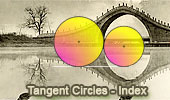Online Geometry: Tangent Circles, Theorems and Problems, Page 7

 Tangent Circles, Theorems and Problems: Table of Content 7/8Circumcircles or circumscribed circle Proposed Problem 93. Similar Triangles, Circumcircles, Parallelogram. Tangent circles. Circumcircles or circumscribed circle Proposed Problem 92. Similar Triangles, Circumcircles, Circumradii, Parallel. Feuerbach Points and Nine-Point Circle with interactive animation, manipulation, and step-by-step construction. Tangent circles. Three Tangent Circles Theorem Using TracenPoche Dynamic Software Step-by-Step construction, Manipulation, and animation. Arbelos, Inscribed Circle and Concyclic Points Cyclic Quadrilateral. Tangent circles. Proposed Problem 57: Angle bisector, circles Cyclic Quadrilateral. Tangent circles. Proposed Problem 56: Angle bisector, circles Parallel Lines. Tangent circles. Proposed Problem 55: Angle bisector, circles Cyclic Quadrilateral. Tangent circles. Proposed Problem 54: Angle bisector, circles Midpoint of arc. Tangent circles. Go to page: Previous | 1 | 2 | 3 | 4 | 5 | 6 | 7 | 8 | Next